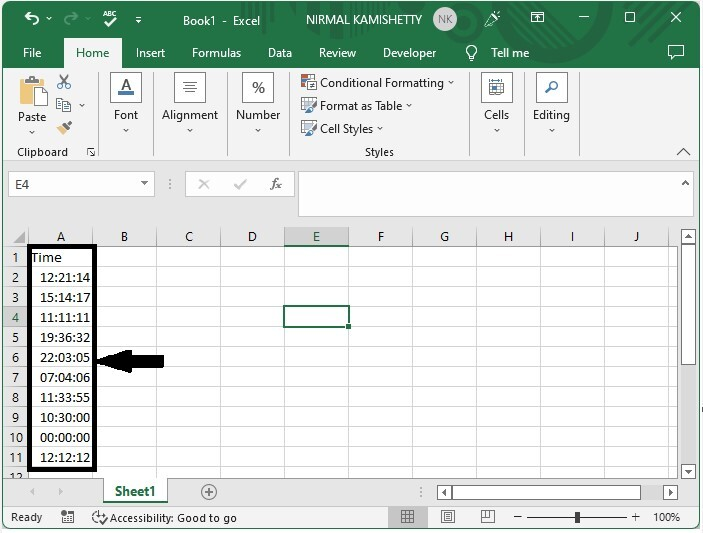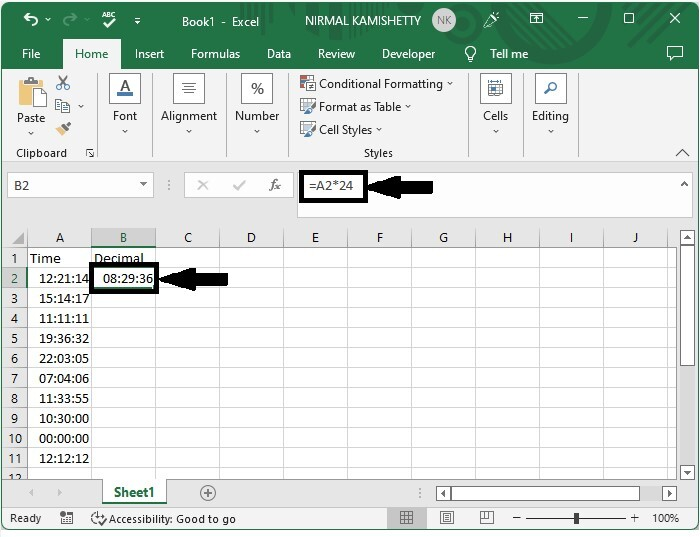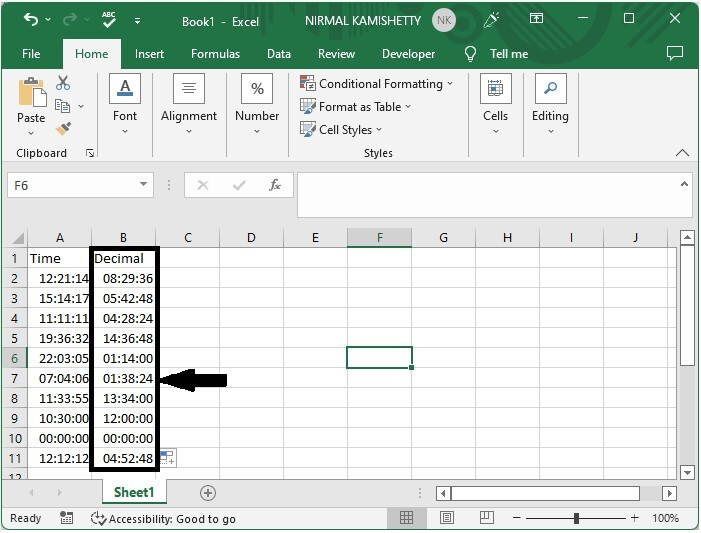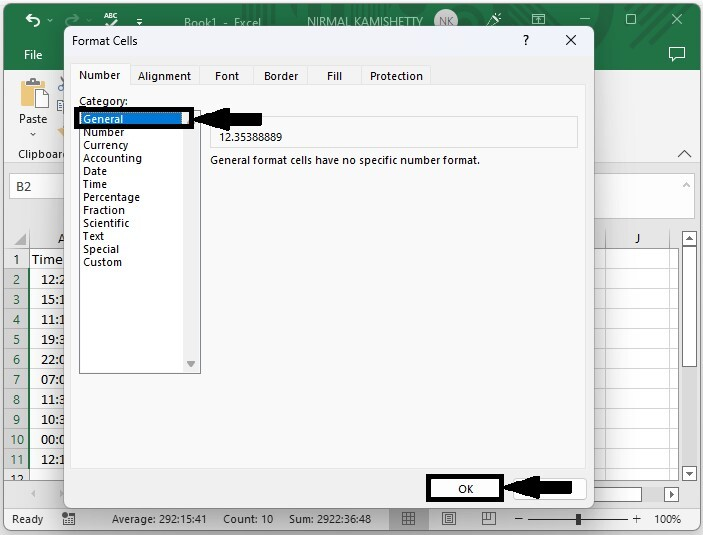# How to Convert Hours, Minutes and Seconds to Decimal Hours in Excel?

Decimal time is time that is represented as a number. In Excel, you may have needed to convert the given time to decimal values at times. If we try to do this task manually, then it can be a time-consuming process. We can use the faster approach mentioned in this article. We can solve this task using the formulas supported in Excel. In this tutorial, we will learn how to convert hours, minutes, and seconds to decimal hours in Excel. This process has two steps: first, converting the time to hours, then formatting the cells.

## Convert Hours, Minutes and Seconds to Decimal Hours

Here, we will first convert the data into hours using the formula and then use the format to convert the time format into decimals. Let us see a simple process to know how we can convert hours, minutes, and seconds to decimal hours in Excel.

### Step 1

Let us consider an excel sheet where the data sheet contains a list of times in hours, minutes, and seconds, similar to the below image.Then, in our case, click on an empty cell, B2, and enter the formula =A2*24, then press Enter to get our first result. In the formula, A2 is the address of a value on the sheet.

Empty cell > formula > enter### Step 2

Then drag down from the first result using the auto-fill handle to get all the values as shown in the below image.Now we need to convert those values in time to decimals.

### Step 3

To do so, select the values, then right-click and select format cells; in the pop-up window, click on "general" and click "ok" to complete our process.

Select data > right click > format cells > general > ok## Conclusion

In this tutorial, we used a simple example to demonstrate how you can convert hours, minutes, and seconds to decimal hours in Excel.

Updated on: 06-Mar-2023

1K+ Views